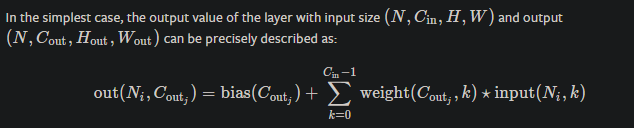# Convolving a 2D Kernel on each channel?

I have a single 2D Kernel of size [3,3], and a Tensor of size [B, 64, H, W]

How can I apply this 2D kernel on each channel?

How should i reshape/repeat the kernel?

I tried to repeat my Kernel, as follows:

`kernel = kernel.repeat((B, 64, 1, 1))`

But when I apply it the tensor size changes

`[1, 64, H, 1]`

I am forced to do this for now but it is inefficient:

``````    for b in range(0, dpv_permuted.shape):
for c in range(0, dpv_permuted.shape):
``````

Hi,

Here is the issue

If your input size is `[64, h, w]` (batch_size does not matter), and if you want to have outputs with size `[64, new_h, new_w]`, then you need 64 filters which each one is [64, 3, 3]. Literally a `[64, 64, 3, 3]` filter is needed.

``````
b = 2
c = 64
h, w = 15, 15

x = torch.randn(b, c, h, w)
kernel = (1/9)*torch.tensor([[1, 1, 1],
[1, 1, 1],
[1, 1, 1]])
kernel = kernel.repeat((64, 64, 1, 1))
print(kernel.shape)  # torch.Size([64, 64, 3, 3])
print(res.shape)  # torch.Size([2, 64, 15, 15])
``````

Bests

I tried your code, but it gives me completely different results. Here are my two functions:

``````spread_kernel = None
dpv_permuted = dpv.permute(0, 3, 2, 1)

kernel = torch.Tensor(np.zeros((N, N)).astype(np.float32))
kernel[int(N / 2), :] = 1 / float(N)
kernel = kernel.repeat((dpv_permuted.shape, dpv_permuted.shape, 1, 1)).to(dpv_permuted.device)

dpv_permuted = F.conv2d(input=dpv_permuted, weight=kernel, padding=N // 2)

dpv = dpv_permuted.permute(0, 3, 2, 1)
tofuse_dpv = dpv / torch.sum(dpv, dim=1).unsqueeze(1)

# torch.Size([128, 384])
# torch.Size([1, 1, 5, 5])
dpv_permuted = dpv.permute(0, 3, 2, 1)
kernel = torch.Tensor(np.zeros((N, N)).astype(np.float32))
kernel[int(N / 2), :] = 1.
print(kernel)
# kernel[2,2] = 1.
kernel = kernel.unsqueeze(0).unsqueeze(0)
kernel = kernel.repeat((1, 1, 1, 1))
print(kernel.shape)
kernel = {'weight': kernel.to(dpv_permuted.device), 'padding': N // 2}

for b in range(0, dpv_permuted.shape):
for c in range(0, dpv_permuted.shape):

dpv = dpv_permuted.permute(0, 3, 2, 1)
tofuse_dpv = dpv / torch.sum(dpv, dim=1).unsqueeze(1)
``````

They are suppose to be equivalent in theory. But both give completely different output

1 Like

Sorry for late answer, here is the idea.
If you look up the definition of multi-channel cross-correlation which is also available in Conv2d docs, you can see below formula:It says, for each output channel, you need to combine correlation results using sum. In your code, you have removed the correlation between different input channels.
Let’s talk intuitively.
If we have an input tensor `X` with size `[in_channel, h, w]` and we want to have 3 output channels for the result `[3, h, w]`, then we need to convolve `x` with a `[3, k, k]` kernel 3 times and concatenate the results. But here is the idea, if kernel is same for all the three times, should not the result also be same as both `x` and `kernel` are identical for each output channel? The answer would be yes which can be achieved by that summation, if we remove that we break this.

Here is your code with some modifications:

``````
torch.manual_seed(0)

kernel_ = None
global kernel_

dpv_permuted = dpv.clone()
kernel = torch.Tensor(np.zeros((N, N)).astype(np.float32))
kernel[int(N / 2), :] = 1.
kernel = kernel.repeat((dpv_permuted.shape, dpv_permuted.shape, 1, 1)).to(dpv_permuted.device)
kernel_ = kernel
dpv_permuted = F.conv2d(input=dpv_permuted, weight=kernel, padding=N // 2)

dpv = dpv_permuted
return dpv

dpv_permuted = dpv.clone()
kernel = torch.Tensor(np.zeros((N, N)).astype(np.float32))
kernel[int(N / 2), :] = 1.
kernel = kernel.unsqueeze(0).unsqueeze(0)
kernel = kernel.repeat((1, 1, 1, 1))
kernel = {'weight': kernel.to(dpv_permuted.device), 'padding': N // 2}

for b in range(0, dpv_permuted.shape):
for c in range(0, dpv_permuted.shape):

dpv = dpv_permuted
return dpv

x = torch.randint(1, 3, (1, 3, 7, 7)).float()
``````

modifications:

1. PyTorch uses channel first conv, so you should remove `.permute` lines unless explicitly tell conv that is channel last.
2. For simplicity I removed normalization parts.
3. You were creating different kernel for first method by dividing `/ float(N)` which had not been used for second method.

If you take `sh` which is the output of your method, then sum wrt channels you will get a output channel for first dimension of `s`.

``````np.sum(sh.numpy(), axis=(0,1))
``````

Also, we can express it by summing the way I initalized kernel:

``````
``````

I tried your modified code and it still doesnt work. It needs to be a drop in replacement for my spread_dpv_hack method. I dont want there to be any correlation between input channels.

Lets simply my problem. Imagine you have a 100 images that you want to apply a 3x3 blur filter. And those 100 images are in a batch of 6. So you have [6,100, r, c]. I just want to apply the 3x3 blur filter across batches and channels. Why is this so difficult

Hi,
If I’m not wrong, you have an input of `[6,100,h,w]` and you want to apply 100 such 3x3 kernels on them such that each Kernel will be applied on only one channel, this leading to output size of `[6,100,h,w]`.
Right ?
If so, you can use this
`nn.Conv2d(in_channels=100, out_channels=100, groups=100, kernel_size= (3,3))`

2 Likes

Ok,
I thought you still looking to hold correlation between channels, now I understand. In this case @chetan_patil 's explanation is the correct way. Sorry about my misinterpretation.Can you please provide some example code

Hello @soulslicer, here it is.

``````ip = torch.randn(6, 100, 8, 10)
conv = nn.Conv2d(in_channels=100, out_channels=100, groups=100, kernel_size= (3,3))
op = conv(ip)
print(op.shape)
torch.Size([6, 100, 6, 8])

``````